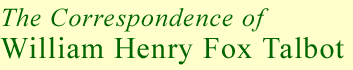Project Director: Professor Larry J Schaaf
 Back to the letter search > Result number 5 of 12:   < Back     Back to results list   Next >   Document number: 6373Date: 13 Jan 1866 Postmark: 13 Jan 1866Recipient: TALBOT William Henry FoxAuthor: TAIT Peter Guthrie Collection: British Library, London, Manuscripts - Fox Talbot Collection Collection number historic: Acc 38761 Last updated: 8th November 2012

6 Greenhill Gardens,
13 / 1 / 66

My dear Sir,

Suppose á to be a prime factor of x – other than m – Then since

xm = ym + zm = y + z {y + zm-1 + mQy + z + mzm-1}

(as is easily seen by putting (y + z - z) for y) if á divide y + z, á m must do so, else á would divide m or z, which it is supposed not to do.

Hence, still supposing x not m, all its factors prime or not which divide y + z do so m times. If, then, A be their product, B that of the other factors,

x + AB & y + z = Am

ym + zm = xm = AmBm.

Hence

Am(Bm - 1) = (ym - y) + (zm - z).

Now, by a theorem of Fermat’s,

î m - î is always divisible by m. Hence Am(Bm - 1) is so divisible – but A is not, by hypothesis, so Bm - 1 is; i.e. (Bm - B) + (B - 1) is divisible by m – i.e. B = mC + 1.

I know very little about the theory of numbers, having only glanced over Legendre and Barlow: and probably in consequence of defective knowledge, I have wasted much time on Fermat’s, and other, theorems. I thought I had proved that A also is of the form Cm + 1; in which case x would be of the same form – and the same reasoning would apply to one of the two y & z. The third would obviously be divisible by m. Thus it would be reduced to showing the impossibility of

(Pm + 1)m = (Qm + 1)m + Rmmm.

But the proof involved a fallacy (common to such investigations) & I am not sure that the above is true.

The above gives, however, a very simple proof of the theorems you tell me are found in Barlow & Legendre.

Yours (in very great haste)
P. G. Tait.

H. F. Talbot Esqre

[envelope:]
H. F. Talbot Esqre
13 Great Stuart Street,
(Edinh)

P.G. Tait

 Result number 5 of 12:   < Back     Back to results list   Next >